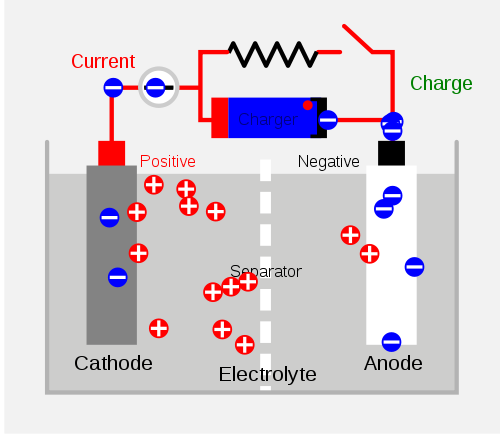# Rechargeable Batteries

$$\newcommand{\vecs}{\overset { \rightharpoonup} {\mathbf{#1}} }$$ $$\newcommand{\vecd}{\overset{-\!-\!\rightharpoonup}{\vphantom{a}\smash {#1}}}$$$$\newcommand{\id}{\mathrm{id}}$$ $$\newcommand{\Span}{\mathrm{span}}$$ $$\newcommand{\kernel}{\mathrm{null}\,}$$ $$\newcommand{\range}{\mathrm{range}\,}$$ $$\newcommand{\RealPart}{\mathrm{Re}}$$ $$\newcommand{\ImaginaryPart}{\mathrm{Im}}$$ $$\newcommand{\Argument}{\mathrm{Arg}}$$ $$\newcommand{\norm}{\| #1 \|}$$ $$\newcommand{\inner}{\langle #1, #2 \rangle}$$ $$\newcommand{\Span}{\mathrm{span}}$$ $$\newcommand{\id}{\mathrm{id}}$$ $$\newcommand{\Span}{\mathrm{span}}$$ $$\newcommand{\kernel}{\mathrm{null}\,}$$ $$\newcommand{\range}{\mathrm{range}\,}$$ $$\newcommand{\RealPart}{\mathrm{Re}}$$ $$\newcommand{\ImaginaryPart}{\mathrm{Im}}$$ $$\newcommand{\Argument}{\mathrm{Arg}}$$ $$\newcommand{\norm}{\| #1 \|}$$ $$\newcommand{\inner}{\langle #1, #2 \rangle}$$ $$\newcommand{\Span}{\mathrm{span}}$$$$\newcommand{\AA}{\unicode[.8,0]{x212B}}$$

Rechargeable batteries (also known as secondary cells) are batteries that potentially consist of reversible cell reactions that allow them to recharge, or regain their cell potential, through the work done by passing currents of electricity. As opposed to primary cells (not reversible), rechargeable batteries can charge and discharge numerous times.

## Introduction

Secondary cells encompass the same mechanism as the primary cells with the only difference being that the Redox reaction of the secondary cell could be reversed with sufficient amount of energy placed into the equation. The figure below illustrates the mechanism of a charging secondary cell. The Charger shown on the top of the diagram is pulling the negative charges toward the right side of the separator. This makes it seem like the positive charges are compiling on the other side of the cell which is not allowed to pass the separator. This disequilibrium is the representation of the cell potential that, when allowed, could once again approach equilibrium through the transferring of the electrons.Different secondary batteries provide various functions. For long-term use (followed by discharging and charging), long storage time when not in use, remote activation, and use under harsh weather conditions are just a few obstacles of creating such secondary cells. Unfortunately, there are no batteries that are capable of encompassing all functions mentioned above. Therefore, the user must decide which application is the most important for a specific task in order to determine the most compatible version of rechargeable batteries.

Lead-acid batteries are one of the most common secondary batteries, used primarily for storing large cell potential. These are commonly found in automobile engines. Its advantages include low cost, high voltage and large storage of cell potential; and disadvantages include heavy mass, incompetence under low-temperatures, and inability to maintain its potential for long periods of time through disuse. The reactions of a lead-acid battery are shown below:

• cathode (reduction):

$PbO_{2(s)} + HSO^−_{4(aq)} + 3H^+_{(aq)} + 2e^− \rightarrow PbSO_{4(s)} + 2H_2O_{(l)} \label{19.90}$

• anode (oxidation):
$Pb_{(s)} + HSO^−_{4(aq)} \rightarrow PbSO_{4(s) }+ H^+_{(aq)} + 2e^−\label{19.91}$
• overall:

$Pb_{(s)} + PbO_{2(s)} + 2HSO^−_{4(aq)} + 2H^+_{(aq)} \rightarrow 2PbSO_{4(s)} + 2H_2O_{(l)} \label{19.92}$

Discharging occurs when the engine is started and where the cell potential equals 2.02V. Charging occurs when the car is in motion and where the electrode potential equals -2.02V, a non- spontaneous reaction which requires an external electrical source. The reverse reaction takes place during charging.

The nickel-cadmium (NiCd) battery is another common secondary battery that is suited for low-temperature conditions with a long shelf life. However, the nickel-cadmium batteries are more expensive and their capacity in terms of watt-hours per kilogram is less than that of the nickel-zinc rechargeable batteries.

• cathode (reduction):

$2NiO(OH)_{(s)} + 2H_2O_{(l)} + 2e^− \rightarrow 2Ni(OH)_{2(s)} + 2OH^-_{(aq)} \label{19.86}$

• anode (oxidation):

$Cd_{(s)} + 2OH^-_{(aq)} \rightarrow Cd(OH)_{2(s)} + 2e^- \label{19.87}$

• overall:

$Cd_{(s)} + 2NiO(OH)_{(s)} + 2H_2O_{(l)} \rightarrow Cd(OH)_{2(s)} + 2Ni(OH)_{2(s)} \label{19.88}$

Advantages of the nickel-zinc battery are its long life span, high voltage, and the sufficient energy to mass to volume ratio. These characteristics make the nickel-zinc battery more attractive than some. However, it is not yet made in sealed form.